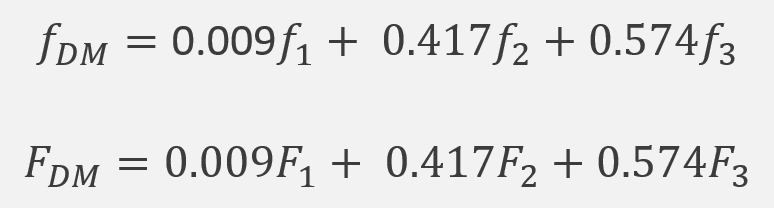# 3.4.1 The Decision Maker

Course subject(s) Module 3. Performance-based weights and the Decision Maker

The performance-based weights allow use to combine experts assessments. More specifically, they allow us to combine experts’ distributions.

The obtained distribution is that of a Decision Maker and is given by the following formulaswhere recall that denotes the probability density function(pdf) and F denotes the cumulative distribution function (cdf).

For the Dutch eating habits example, we assume w1=0.009, w2=0.417 and w3=0.574.

The probability density function (pdf) and the cumulative distribution function (cdf) of the Decision Maker (DM) are obtained fromWithin the Classical Model, the term Decision Maker (DM) is used to refer to the resulted combined distribution; the DM would corresponds to a virtual expert.

It is assumed that the real decision maker would adopt this distribution as their own.